Clairaut's theorem on equality of mixed partials

Statement

Statement for second-order mixed partial of function of two variables

Suppose$f$ is a real-valued function of two variables$x,y$ and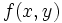$f(x,y)$ is defined on an open subset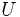$U$ of$\R^2$. Suppose further that both the second-order mixed partial derivatives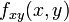$f_{xy}(x,y)$ and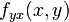$f_{yx}(x,y)$ exist and are continuous on$U$. Then, we have:$\! f_{xy} = f_{yx}$

on all of$U$.

General statement

The statement can be generalized in two ways:

• We can generalize it to higher-order partial derivatives.
• We can generalize it to functions of more than two variables.

The general version states the following. Suppose$f$ is a function of$n$ variables defined on an open subset$U$ of$\R^n$. Suppose all mixed partials with a certain number of differentiations in each input variable exist and are continuous on$U$. Then, all the mixed partials are continuous.

Some examples are given below:

• Suppose$f$ is a function of two variables$x$ and$y$, and the three mixed partials$f_{xxy}, f_{xyx}, f_{yxx}$ exist and are continuous on an open subset$U$ of$\R^3$. Then, all three of them are equal on$U$. (Note that these mixed partials all involve differentiating twice with respect to$x$ and once with respect to$y$).
• Suppose$f$ is a function of three variables$x,y,z$, and the six mixed partials$f_{xyz}, f_{xzy}, f_{yxz}, f_{yzx}, f_{zxy}, f_{zyx}$ exist and are continuous on an open subset$U$ of$\R^3$. Then, all six of them are equal on$U$.
CAUTION: This general version of Clairaut's theorem does not merely state that if two higher-order mixed partials that involve the same number of differentiations in each variable are continuous, they must be equal. Rather, they require all the higher-order mixed partials of that type to be defined and continuous. The reason is that in the proof of this using Clairaut's theorem for second-order mixed partials, we may need to go via the other higher-order mixed partials and hence need those to exist and be continuous as well. Thus, the generalization does not say, for instance, that if$f_{xxy}$ and$f_{yxx}$ exist and are continuous, they must be equal.
We can formulate a slightly stronger version of this general statement, that only requires the continuity of certain specific other partial derivatives, but this would get too complicated.

Note: The video below will probably be replaced by a better video that covers the subtleties better, but it's provisionally okay.

Particular cases

Clairaut's theorem can be verified in a number of special cases through direct computations. Some of these are illustrated below.

For further information, refer: Additively separable function

Suppose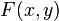$F(x,y)$ is an additively separable function of two variables, i.e., we can write:$\! F(x,y) := f(x) + g(y)$

where$f,g$ are both functions of one variable. Assume that both$f$ and$g$ are both differentiable. Then, we have:$\! F_x(x,y) = f'(x), F_y(x,y) = g'(y)$

Differentiating each of these a second time with respect to the other variable, we obtain that:$\! F_{xy}(x,y) = 0, F_{yx}(x,y) = 0$

Thus, we see that both second-order mixed partial derivatives are the zero function. In particular, they are equal to each other.

Multiplicatively separable functions

For further information, refer: Multiplicatively separable function

Suppose$G(x,y)$ is a multiplicatively separable function of two variables, i.e., we can write:$\! G(x,y) := f(x)g(y)$

where$f,g$ are both functions of one variable. Assume that both$f$ and$g$ are both differentiable. Then, we have: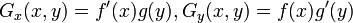$\! G_x(x,y) = f'(x)g(y), G_y(x,y) = f(x)g'(y)$

Differentiating each of these a second time with respect to the other variable, we obtain that:$\! G_{xy}(x,y) = f'(x)g'(y), G_{yx}(x,y) = f'(x)g'(y)$

We thus see that both the second-order mixed partial derivatives are equal.

Note that the proof for multiplicatively separable functions can be easily generalized to functions that are expressible as sums of multiplicatively separable functions. This, therefore, includes all polynomial functions.

Proof

Key computation as a double limit at a point

Based on the definition as a double limit at a point, we have: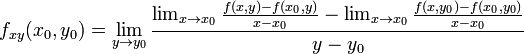$f_{xy}(x_0,y_0) = \lim_{y \to y_0} \frac{\lim_{x\to x_0} \frac{f(x,y) - f(x_0,y)}{x- x_0} - \lim_{x \to x_0} \frac{f(x,y_0) - f(x_0,y_0)}{x- x_0}}{y - y_0}$

Now, conditional to the existence of this expression, we can use that limit is linear to combine the two limits in the inner sum on the right side and get:$f_{xy}(x_0,y_0) = \lim_{y \to y_0} \frac{\lim_{x\to x_0} \left(\frac{f(x,y) - f(x_0,y)}{x- x_0} - \frac{f(x,y_0) - f(x_0,y_0)}{x- x_0}\right)}{y - y_0}$

With some simple algebraic tidying up, this becomes:$f_{xy}(x_0,y_0) = \lim_{y \to y_0} \lim_{x\to x_0} \left[\frac{f(x,y) - f(x_0,y) - f(x,y_0) + f(x_0,y_0)}{(x -x_0)(y - y_0)}\right]$

A similar calculation for the other mixed partial yields that: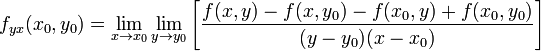$f_{yx}(x_0,y_0) = \lim_{x \to x_0} \lim_{y\to y_0} \left[\frac{f(x,y) - f(x,y_0) - f(x_0,y) + f(x_0,y_0)}{(y -y_0)(x - x_0)}\right]$

We notice that, although the terms are written in a slightly different order, the expressions whose limit is being computed is the same in both cases. The only difference between the two cases is the order in which we are taking limits. In the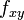$f_{xy}$ case, we take the limit with respect to$x \to x_0$ on the inside, and then take the limit with respect to$y \to y_0$ on the outside. In the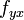$f_{yx}$ case, we take the limit with respect to$y \to y_0$ on the inside, and then take the limit with respect to$x \to x_0$ on the outside.